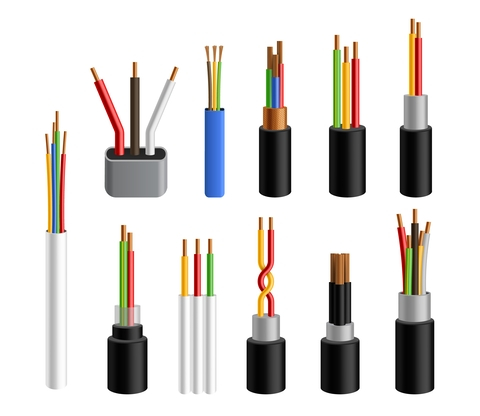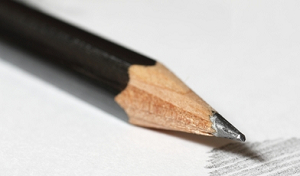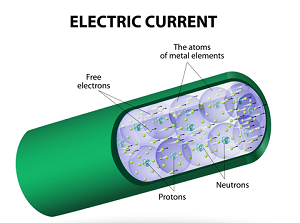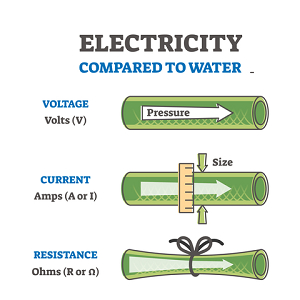# Electric Currents In Conductors

## Introduction

Electric current and conductors are essential for each other because the flow of current is only possible in conductors without any loss. The current is a flow of charges in a particular direction. The flow of current through a conductor is possible due to the difference in potential at both ends.A conductor also charges particles whether it is connected to a battery or not. However, when it is not related to any source of voltage then charges are just neutral and moving in random directions which results in net-zero charge flow and thermal velocity. So, to get a net electric current through a conductor, we apply a battery with two terminals positive and negative. Hence, due to potential differences, the charges start flowing from high potential to low potential

## What is an Electrical Conductor?

In simple words, the object that works as a bridge or connection is the conductor. A material that can be used to conduct electricity is a conductor. The material which allows the electric current to flow through them is called an Electric conductor. The flow of electric charge through the conductor is due to electrons present on the outer shell of atoms. These are free electrons that move freely in a conductor when the conductor is not connected to the battery.

As soon as we apply external voltage with the help of a battery or another power source. Due to potential differences, the charged particles, such as electrons; start drifting from negative to positive. This drifting of all electrons produces electricity in the opposite direction of the drifting of the electron.

The conductors are of various types and classified based on their raw material.• Most conductors we use in our daily life are made of metal like silver, copper, etc. These are called Metal Conductors.

• Some of the conductors in laboratory or experimental fields are constructed of Carbon. Here we use graphite.

• In the liquid form of conductor, we use ionic-conductors, such as saltwater.

## Insulators

An insulator has all the opposite properties of a conductor. The Insulator can be defined as the material which does not allow an electric current to pass through and does not connect to electricity. The insulators have high resistivity and low connectivity due to the high band energy gap between the valence bond and the conduction band.

If we are looking for conduction then insulators are not so beneficial for us but for preventing unwanted conduction that causes electricity loss we can use insulators. Also, insulators are used by workers who work in the electrical engineering field, such as insulating coating on handles, insulating gloves, etc. All the insulators are made of rubber, wood, plastic, stone, etc. There are few most operational commercial insulators are the following −

• Pin Insulator

• Disc Insulator

• Suspension Insulator

• Strain Insulator

• Shackle insulator

## Effect on the Flow of Electrons

The flow of electrons in the conductor is affected by several factors.

• Resistance of Conductor: The flow of electrons in the conductor and circuit is directly inversely proportional to the resistance of the conductor. Thus, the Higher the resistance lower will be electron flow.

• The amount of current also affects the flow of electrons. If there is 1 Ampere current passing through the conductor has fewer electrons than the conductor having 2 Ampere current. The amount of current is directly proportional to an applied electric field.

## What is Current?

Electric current can be defined as the flow of a group of charged particles, such as electrons, ions, etc in a particular direction with the influence of any force like the potential difference. Electric current is the amount of charge flow through a conductor of a unit cross-section area in unit time. The SI unit of electric current is Ampere which is equal to one coulomb per second.

## Visualizing Electric Current

As electric current flow is somewhat doubtful to understand due to some technical terms like potential difference, resistance, etc. For a better and clear understanding, we can use the below diagram.Here, we can see that the pipe is full of water, same as these pipe conductors are also full of charged particles. But the flow of water is only possible with the help of an external source pressure. Now, the same process happens in the flow of electric current. In an electric circuit, external pressure is the potential difference, control nob is resistance, water molecules are charged particles and pipe is the conductor.

## The Direction of the Current

The direction of the current is not so well-known by people. Mostly, books skipped this topic due to the little and doubtful information the authors have.In general, we think that the direction of current is from higher potential to low means from a positive terminal to negative terminal which is known as conventional current and also the direction of current for maximum people.

However, when we study electric current deeply we find that the current is the flow of charged particles in the conductor, and conductors' electrons are free to move under the influence of external force. Hence, electrons are negatively charged and they move from negative to positive. So, the flow of electrons from negative to positive in the direction of the current.

## Current in the Conductors

In a conductor, there are millions of free electrons which move in any random direction without any influence of an external electric field or force. Every electron is in a different direction so their net velocity of zero in opposite direction cancels each other.

However, when we apply any field to the conductor, it aligns all the free electrons in a constant direction and starts moving due to the influence of electrostatic force. The electrons are negatively charged so they start moving in a positive direction.

This produces a continuous flow of charged particles known as electric current.

## Mobility of an Electron

In simple words, mobility means the capability to move. We all know that free-charged particles in conductors move when it comes under the influence of an external source of force. When these charge carriers are moving freely there is net-zero drift velocity but with the impact of the externally applied field charged particles start drifting in the conductor according to the nature of their charge. This drifting has a particular velocity according to the strength of the applied field.

Hence, mobility can be defined as the drift velocity of electrons or any other charge carrier per unit electric field that is applied externally.

The notation of Mobility is $\mathrm{\mu}$

$$\mathrm{Mobilty(\mu )=\frac{v}{E}}$$

Here, V = Velocity of charged particles

E = the applied electric field

## Importance of Electric Current in Conductors

The electric current is very important for conductors and electric devices. Some of the importance of electric current in conductors are below.

• As most of the devices in our homes are electric powered so current is very important in conductors for our balanced-daily lifestyle.

• If there will be no current in the conductor then the world will not be able to develop and manage the demand or supply chain that will affect living on earth.

• Also, without current in a conductor, we will not able to make communication with our relatives and friends.

• Modern transportation is also powered by an electric current that will be also affected if there will be no current in conductors.

## FAQs

Q1. What are good electric conductors?

Ans. Good electric conductors are those which have high conductivity and low resistivity. Also, some of the conductors which are built of copper, gold, and silver are the best electrical conductors.

Q2. What are semi-conductors?

Ans. The conductors which are not pure conductors nor pure insulators are semiconductors. The conductivity of semiconductors lies between the insulators and conductors. Silicon and Germanium are the best examples of semiconductors.

Q3. What do you mean by electric circuit?

Ans. An electric circuit can be defined as a closed and continued path of electric current which include a working device, conductor, and battery.

Q4. What is the effect of the length of the conductor on current flow?

Ans. The length of the conductor is a very important factor that affects the amount of current flow through the conductor. The resistance has a direct relation to length. Hence, lengthy wire offers more resistance to current flow which decreases the overall amount of current flow through the conductor.

Q5. What is Drift Velocity?

Ans. When we place a conductor under the influence of an electric field, the free charge carriers will move under field influence. This movement is called drifting. Hence, the average velocity of this drifting of charged particles is called drift velocity.

Updated on: 24-Jan-2023

1K+ Views